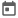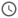# Different types of loops in Verilog##### 13 Sep 2021##### 4 mins

Loops are beneficial as it makes it easy to do repetitive work. Also, loops iterate through different elements of an array and process them. Verilog provides many types of loops that are beneficial in certain use cases. Different loop constructs present in Verilog are:

1. For loop
2. While loop
3. Forever loop
4. Repeat loop

Let us see different loop constructs of Verilog in detail.

### For Loop

For loop is used when we want to stop loop iteration depending on some condition. For loop may not execute even once if the exit condition is valid for the first iteration. In simpler terms, before executing each iteration, the loop checks the condition, and if the condition is false, the loop exits.

Syntax: `for(<initial value>;<condition>;<update_rule>) begin end`

In Verilog, we cannot declare the iterator inside a loop. Iterator is nothing but the variable used to implement the loop, variable `i` in this case. Also, as `i++` is not valid in Verilog, we need to use `i=i+1` to increase the variable's value by 1.

From the design point of view, for loop, is used to replicate particular hardware.

``````module for_loop;
// declare a loop iterator
integer i;

initial begin
for (i=0; i<5; i=i+1) begin
\$display("3 * %0d = %0d", i, 3*i);
end
end
endmodule``````
##### Output
``````# 3 * 0 = 0
# 3 * 1 = 3
# 3 * 2 = 6
# 3 * 3 = 9
# 3 * 4 = 12``````

### While Loop

While loops are used when the number of iterations is indeterminate, and exit from the loop is based on condition. Also, in this case, the iterator is not available directly, and any variable can be used to write the condition. `while` loop is different from `for` loop as in while loop, we need to give the exit condition inside the loop construct, and thus gives us the flexibility in defining variable update rule.

Syntax: `while(<condition>) begin end`

``````module while_loop;
// declare a normal variable
reg[7:0] i;

initial begin
i = 0; // initializing the iterator with default value
while(i < 5) begin
\$display("3 * %0d = %0d", i, 3*i);
i = i + 1;
end
end
endmodule``````
##### Output
``````# 3 * 0 = 0
# 3 * 1 = 3
# 3 * 2 = 6
# 3 * 3 = 9
# 3 * 4 = 12``````

### Forever Loop

A forever loop is an infinite loop, i.e., the loop continues to run infinitely. Forever loop is mainly used when we want some code block to run infinitely, basically in a test bench. It should be used with caution as this can lead to ever ending simulations, as by default, a forever loop blocks the execution of the code until the loop is ended. Forever loop should be used inside the fork-join construct to run it concurrently. It can also be ended using break or return.

Always and forever loop may seem the same, as they both execute the code written inside it infinitely, but it is not the case. As `always` is a procedural block; thus, it is concurrent, as discussed in this article, and synthesizable. However, forever loop is a loop construct that is only used inside a procedural block, i.e., `initial` or `always` block, and by default, the loop blocks the execution of the rest of the code until it gets out of the loop.

Syntax: `forever begin end`

#### Example 1

In this example, the `forever` loop is used inside an `initial` block, and in another `initial` block, `\$finish` is called at the `10th time step`. As there are two initial blocks, they will run concurrently, and the simulation will stop at t=10. However, if the delay of one unit is removed from the `forever` loop, the simulation would never end. Because when the delay is removed from the forever loop, the simulation will never proceed, and the loop will run forever. `\$fnisih` could not be called in this case. Try removing the delay and see the output.

``````module forever_loop_1;
initial begin
forever begin
\$display("[%0t] Iterating", \$time);
#1;
end
end
initial begin
#10;
\$finish;
end
endmodule``````
##### Output
``````#  Iterating
#  Iterating
#  Iterating
#  Iterating
#  Iterating
#  Iterating
#  Iterating
#  Iterating
#  Iterating
#  Iterating
# ** Note: \$finish    : loops.v(35)
#    Time: 10 ns  Iteration: 0  Instance: /forever_loop_1``````
Try this code in EDA Playground

#### Example 2

In this example, the `forever loop` is terminated using break. As break is not directly available in Verilog, thus `disable` keyword is used to achieve it. A detailed explanation can be found here. Removing the `disable` statement will make the simulation run indefinitely.

``````module forever_loop_2;
integer i;
initial begin: loop
i = 0;
forever begin
\$display("3 * %0d = %0d", i, 3*i);
i = i + 1;
// break if i is greater than 5
if(i > 5)
disable loop;
end
end
endmodule``````
##### Output
``````# 3 * 0 = 0
# 3 * 1 = 3
# 3 * 2 = 6
# 3 * 3 = 9
# 3 * 4 = 12
# 3 * 5 = 15``````
Try this code in EDA Playground

### Repeat loop

A repeat loop does not have an iterator and inputs a number. This simple loop construct repeats the code inside it for the number of times mentioned in the loop construct. It can be seen as the finite variant of the forever loop.

Syntax: `repeat(<no_of_loops>) begin end`

``````module repeat_loop;
initial begin
repeat(5) begin
\$display("Inside loop");
end
\$display("Loop Ended");
end
endmodule``````
##### Output
``````# Inside loop
# Inside loop
# Inside loop
# Inside loop
# Inside loop
# Loop Ended``````